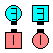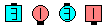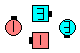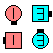Definitions of Square Dance Calls and Concepts

Index -->  Plus  |  A1  |  A2  |  C1  |  C2  |  C3A  |  C3B  |  C4  |  NOL  |
Definitions (Text Only) -->  Plus  |  A1  |  A2  |  C1  |  C2  |  C3A  |  C3B  |  C4  |  NOL  |
 Find call:

 \$B8@8l(B view (admin)

\$BE,@Z\$J(B formation \$B\$+\$i!%(B

Square Thru n To A Wave \$B\$r\$7(B, anything call \$B\$r\$7\$F(B, Step & Fold \$B\$r\$7\$^\$9!%(B

\$B\$\$\$m\$\$\$m\$J(B formations \$B\$G=*\$o\$j\$^\$9!%(B

\$BCm
• anything call \$B\$O(B 8 \$B?M\$N%3!<%k\$,2DG=\$G\$9!%(B
• \$B\$b\$7(B anything call \$B\$,(B 'Lock' \$B\$@\$C\$?\$i(B Lockit \$B\$r\$7(B, anything call \$B\$,(B 'Top' \$B\$@\$C\$?\$i(B Fan The Top \$B\$r\$7\$^\$9!%(B
• \$B;d\$?\$A\$N0U8+\$G\$9\$,(B, \$B%3!<%i!<\$O(B anything call \$B\$KBP\$7\$F>o\$K40A4\$JL>A0\$r;H\$&\$Y\$-\$G\$9!%(B Catch anything n \$B\$O3P\$(\$?\$1\$I(B, Catch Top n \$B\$N(B 'Top' \$B\$,(B (Spin The Top, Relay The Top, Tag The Top, \$B\$J\$I\$N%3!<%k\$G\$J\$/(B) Fan the Top \$B\$r0UL#\$9\$k\$H\$\$\$&\$3\$H\$rCN\$i\$J\$\$?M\$,\$\$\$^\$7\$?!%(B
• anything call \$B\$O(B Diamond \$B\$G=*\$o\$k\$b\$N\$b2DG=\$G\$9!%(B \$B\$=\$N\$H\$-(B, Diamond \$B\$+\$i\$N(B Step & Fold: Centers \$B\$O(B 1/2 Press Ahead, Points \$B\$O(B T-Bone 2 x 2 \$B\$N(B ends \$B\$K\$J\$k\$h\$&\$K(B Fold \$B\$7\$^\$9!%(B

•Catch Switch To A Diamond 3\$B\$NA0(B Square Thru 3 To A Wave\$B\$N8e(B Switch To A Diamond\$B\$N8e(B Step & Fold\$B\$N8e(B (\$B=*\$o\$j(B)

Split Catch anything n [C3A]: Split Square Thru n To A Wave \$B\$r\$7(B, anything call \$B\$r\$7\$F(B, Step & Fold \$B\$r\$7\$^\$9!%(B

\$B

• Catch Motivate 3: Catch anything 3 \$B\$G(B, anything call \$B\$,(B Motivate \$B\$G\$9!%(B

Eight Chain Thru \$B\$+\$i(B: Square Thru on the 3rd hand, full Motivate \$B\$r\$7\$F(B, Step & Fold \$B\$G\$9!%(B

• Catch 3 Motivate: anything Motivate \$B\$G(B anything call \$B\$,(B Catch 3 \$B\$G\$9!%(B

Facing Lines \$B\$+\$i(B: full Catch 3 \$B\$r\$7\$F(B, Finish Motivate (\$B\$9\$J\$o\$A(B, \$B;O\$a\$N(B Circulate \$B\$r>JN,(B) \$B\$G\$9!%(B

• \$B

• Once Removed Catch Mix 3: Once Removed \$B\$G(B, Catch Mix 3 \$B\$N%3!<%k\$r\$7\$^\$9!%(B
• Catch Once Removed Mix 3: \$B8~\$+\$\$9g\$C\$?(B Line \$B\$+\$i(B: Catch anything 3 \$B\$G(B, anything call \$B\$,(B Once Removed Mix \$B\$G\$9!%(B Each side Square Thru but on the 3rd hand, \$BA40w\$G(B Once Removed Mix \$B\$r\$7(B, each side Step & Fold \$B\$r\$7\$^\$9!%(B
•Choreography for Catch anything {n}Comments? Questions? Suggestions?

https://www.ceder.net/def/catchanything.php?language=japan&level=C3A
15-April-2021 11:16:12
Copyright © 2021 Vic Ceder.  All Rights Reserved.